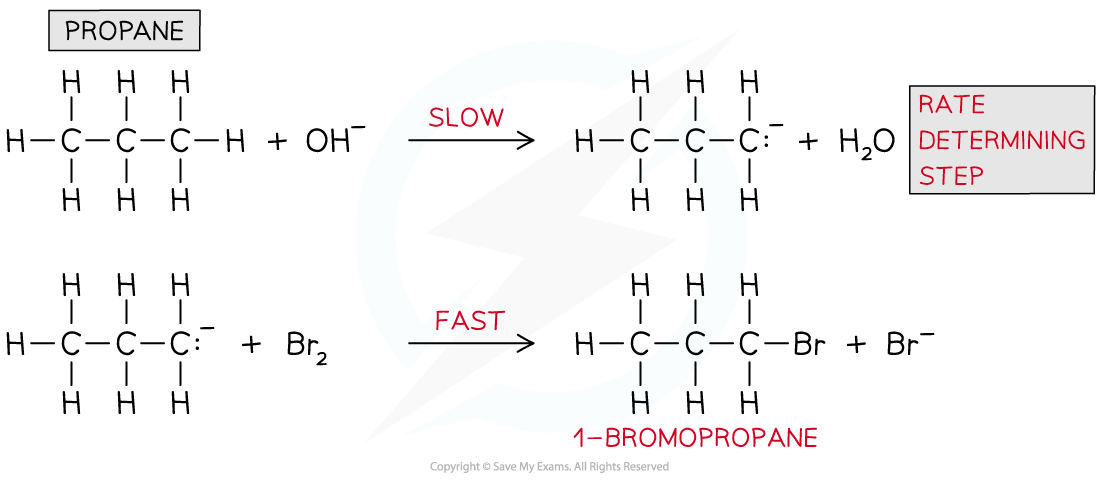# Edexcel A Level Chemistry:复习笔记5.5.7 Rate-Determining Steps

### Deducing Rate-Determining Steps

#### Rate-determining step & intermediates

• A chemical reaction can only go as fast as the slowest part of the reaction
• So, the rate-determining step is the slowest step in the reaction

• If a reactant appears in the rate-determining step, then the concentration of that reactant will also appear in the rate equation
• For example, the rate equation for the reaction below is rate = k [CH3Br] [OH-]

CH3Br + OH- → CH3OH + Br-

• This suggests that both CH3Br and OH- take part in the slow rate-determining step

#### Predicting the reaction mechanism

• The overall reaction equation and rate equation can be used to predict a possible reaction mechanism of a reaction
• This shows the individual reaction steps which are taking place

• For example, nitrogen dioxide (NO2) and carbon monoxide (CO) react to form nitrogen monoxide (NO) and carbon dioxide (CO2)
• The overall reaction equation is:

NO2 (g) + CO (g) → NO (g) + CO2 (g)

• The rate equation is:

Rate = k [NO2]2

• From the rate equation it can be concluded that the reaction is zero order with respect to CO (g) and second order with respect to NO2 (g)
• This means that there are two molecules of NO2 (g) involved in the rate-determining step and zero molecules of CO (g)
• A possible reaction mechanism could therefore be:

Step 1:

2NO2 (g) → NO (g) + NO3 (g)                   slow (rate-determining step)

Step 2:

NO3 (g) + CO (g) → NO2 (g) + CO2 (g)     fast

Overall:

2NO2 (g) + NO3 (g) + CO (g) → NO (g) + NO3 (g) + NO2 (g) + CO2 (g)

=     NO2 (g) + CO (g) → NO (g) + CO2 (g)

#### Predicting the reaction order & deducing the rate equation

• The order of a reactant and thus the rate equation can be deduced from a reaction mechanism if the rate-determining step is known
• For example, the reaction of nitrogen oxide (NO) with hydrogen (H2) to form nitrogen (N2) and water

2NO (g) + 2H2 (g) → N2 (g) + 2H2O (l)

• The reaction mechanism for this reaction is:

Step 1:

NO (g) + NO (g) → N2O2 (g)                      fast

Step 2:

N2O2 (g) + H2 (g) → H2O (l) + N2O (g)     slow (rate-determining step)

Step 3:

N2O (g) + H2 (g) → N2 (g) + H2O (l)           fast

• The second step in this reaction mechanism is the rate-determining step
• The rate-determining step consists of:
• N2O2 which is formed from the reaction of two NO molecules
• One H2 molecule

• The reaction is, therefore, second order with respect to NO and first order with respect to H2
• So, the rate equation becomes:

Rate = k [NO]2 [H2]

• The reaction is, therefore, third order overall

#### Identifying the rate-determining step

• The rate-determining step can be identified from a rate equation given that the reaction mechanism is known
• For example, propane (CH3CH2CH3) undergoes bromination under alkaline solutions
• The overall reaction is:

CH3CH2CH3 + Br2 + OH- → CH3CH2CH2Br + H2O + Br-

• The reaction mechanism is:Reaction mechanism for the bromination of propane under alkaline conditions

• The rate equation is:

Rate = k [CH3CH2CH3] [OH-]

• From the rate equation, it can be deduced that only CH3CH2CH3 and OH- are involved in the rate-determining step and not bromine (Br2)
• CH3CH2CH3 and OH- are only involved in the first step of the reaction mechanism, therefore the rate-determining step is:
• CH3CH2CH3 + OH- → CH3CH2CH2- + H2O